# How to represent piecewise function(cases) in LaTeX?

AMS-LATEX and Mathtools packages provide a means for beautifully typesetting Piecewise-defined functions by rendering large fitting curly brackets either to the left or to the right.

The array package can also be used to beautifully type cases(piecewise-defined functions).

## Piecewise function with cases environment

Below we have the syntax of the cases environment and examples to demonstrate its usage.

\documentclass{article}
\usepackage{amsmath}
\begin{document}
$\begin{cases} ....& \mbox{.....}\\ ....& \mbox{.....}\\ \end{cases}$OR$\begin{cases} ....& \text{.....}\\ ....& \text{.....}\\ \end{cases}$
\end{document}

Examples(Demonstration):

\documentclass{article}
\usepackage{amsmath}
\begin{document}
The absolute value of $x$ is defined
$|x| = \sqrt{x^2} = \begin{cases} x & \mbox{ if  x>0 }\\ 0 & \mbox{ if  x = 0 }\\ -x & \mbox{ if  x< 0 } \end{cases}$
\end{document}

Output :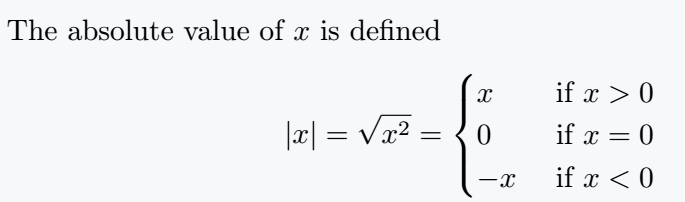The \mbox{...} command create a box and typesets its argument in text mode whose lines are unbreakable.

\text{...} will do the same job but permits line breaks as opposed to mbox{...}.

The & symbol serves the purpose of maintaining the alignment.

## Piecewise function with array environment

To typeset cases using the array environment, we simply make use of curly braces delimiters wherein we restrict one side of the brace and allow the other.

Below we have the syntax of the array environment together with the curly brace and example to demonstrate its usage.

\documentclass{article}
\usepackage{amsmath}
\begin{document}
$\left \{ \begin{array}{column_def} . . . . \\ . . . . \\ . . \end{array}\right.$
OR
$\left. begin{array}{column_def} . . . . \\ . . . . \\ . . \end{array}\right \}$
\end{document}

Examples(Demonstration):

\documentclass{article}
\usepackage{amsmath}
\begin{document}
The sum to infinity of a G.P ($S_{\infty }$) is given by
$S_{\infty} =(S_n)_{n \to \infty } = \left [\frac{a(1-r^n)}{1-r }\right]_{n \to \infty } = \left \{ \begin{array}{l@{\quad} l } \pm \infty ; & \text{ if  | r|>1 }\\ \pm \infty ; & \text{if  r=1 }\\ a ; & \text{if  r=-1  and  n  odd}\\ 0 ; & \text{if  r=-1  and  n  even }\\ \frac{a}{1-r } ; & \text{ if  | r|<1 } \end{array} \right .$
\end{document}

Output :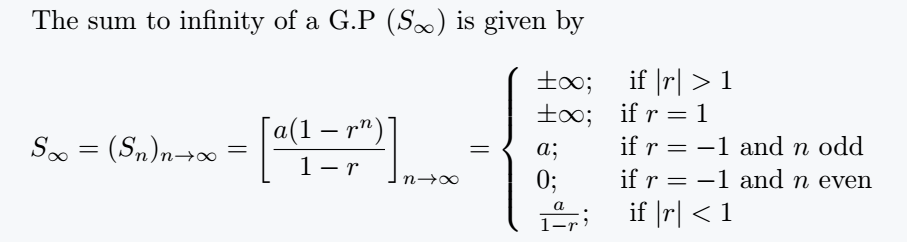The \text{...} command typesets its argument in text mode even when inside the math mode.

This entails that we must deal put a around every symbol or mathematical term. Array column definition is made of up two columns whose alignments are specified to be left(l) and separated by a small horizontal space of 1em with the command @{\quad}. The right curly brace is restricted by using the command \right. as you can see from the code. Same method is applied is the restriction is to be done to the left curly brace. ## Piecewise function with dcase and rcase environment Mathtools provides us with a better single curly bracket environment with a more perfect display. Below we have the following listed environment. \documentclass{article} \usepackage{mathtools} \begin{document} \begin{dcases} & \end{dcases} \begin{dcases∗} & \end{dcases∗} \begin{rcases} & \end{rcases} \begin{rcases∗} & \end{rcases∗} \begin{drcases} & \end{drcases} \begin{drcases∗} & \end{drcases∗} \begin{cases∗} & \end{cases∗} \end{document} Examples(Demonstration): \documentclass{article} \usepackage{mathtools} \begin{document} The sum to infinity of a G.P ( S_{\infty}) $is given by $S_{\infty} = \begin{dcases} \pm \infty ; & \text{ if | r|>1 }\\ \pm \infty ; & \text{if r=1 }\\ a ; & \text{ if r=-1 and n odd}\\ 0 ; & \text{ if r=-1 and n even }\\ \frac{a}{1-r} ; & \text{if | r|<1 } \end{dcases}$ The sum to infinity of a G.P$ ( S_{ \infty }) $is given by $S_{\infty } = \begin{dcases*} \pm \infty ; & \text{ if | r|>1 }\\ \pm \infty ; & \text{ if r=1 }\\ a ; & \text{ if r=-1 and n odd}\\ 0 ; & \text{ if r=-1 and n even }\\ \frac{a}{1-r} ; & \text{if | r|<1 } \end{dcases*}$ \end{document} Output :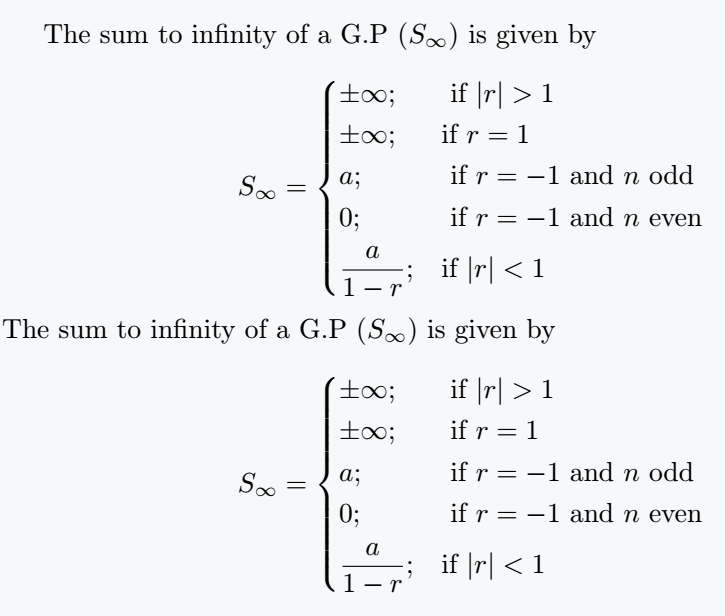You will notice that in the dcases* environment, immediately after & everything if formatted in the text mode therefore there is no need for mbox{...} or \text{...}. \documentclass{article} \usepackage{mathtools} \begin{document} The absolute value of$ x $is defined $\begin{rcases} x & \mbox{if x>0 }\\ 0 & \mbox{ if x = 0 }\\ -x & \mbox{ if x<0 } \end{rcases}= \sqrt{x^2} = | x |$ The absolute value of$ x $is defined $\begin{rcases*} x & if {x>0} \\ 0 & if {x = 0} \\ -x & if {x<0} \end{rcases*}= \sqrt{x^2} = | x |$ \end{document} Output :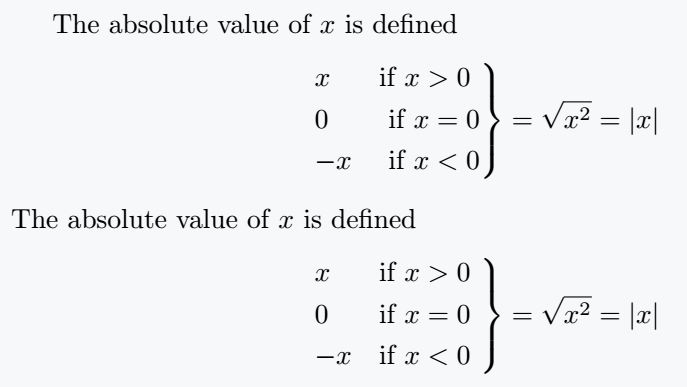Similar explanation except for the fact that there is a change in position of the braces. dcases* and rcases* have and follow the same effects. ## numcases and subnumcases The cases package provides us the numcases and subnumcases environments which permits us to number our equations in our piecewise-defined functions. numcase will number equation different and subnumcases will consider and number as subequations. Below we have the syntax and an example to demonstrate how to go about it. \documentclass{article} \usepackage{amsmath,cases} \begin{document}$\begin{numcases}{...}
.... & \mbox{.....} \\
.... & \mbox{.....} \\
.
.
\end{numcases }$and$\begin{subnumcases}{....}
.... & \text{.....} \\
.... & \text{.....} \\
.
.
\end{subnumcases}\$
\end{document}

Examples(Demonstration):

\documentclass{article}
\usepackage{amsmath,cases}
\begin{document}
$\begin{numcases}{|x|=} x & \mbox{if  x>0 }\\ 0 & \mbox{if  x = 0 }\\ -x & \mbox{if  x< 0 } \end{numcases}$
and
$\begin{subnumcases}{|x|=} x & \mbox{if  x>0 }\\ 0 & \mbox{if  x = 0 }\\ -x & \mbox{if  x< 0} \end{subnumcases}$
\end{document}

Output :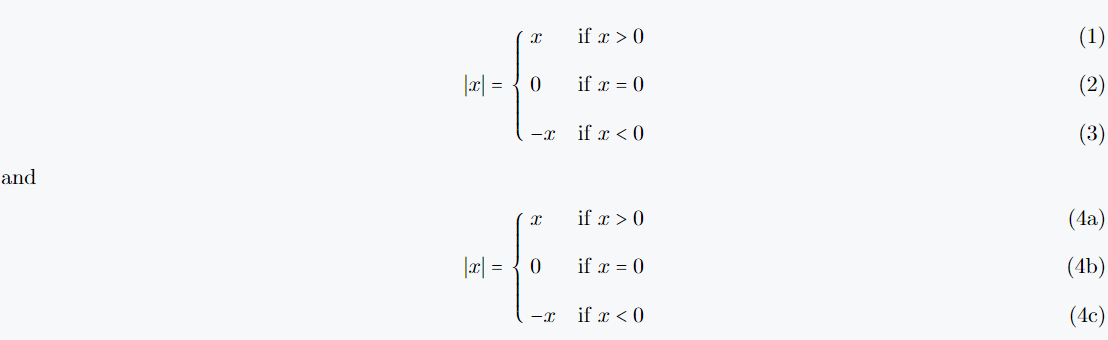## Conclusion

This article gives you full coverage of how to create piecewise-defined functions using various environments.

A good mastery of this tutorial will improve your mathematical typesetting, especially in the domain where this concept is used.#### Md Jidan Mondal

LaTeX expert with over 10 years of experience in document preparation and typesetting. Specializes in creating professional documents, reports, and presentations using LaTeX.